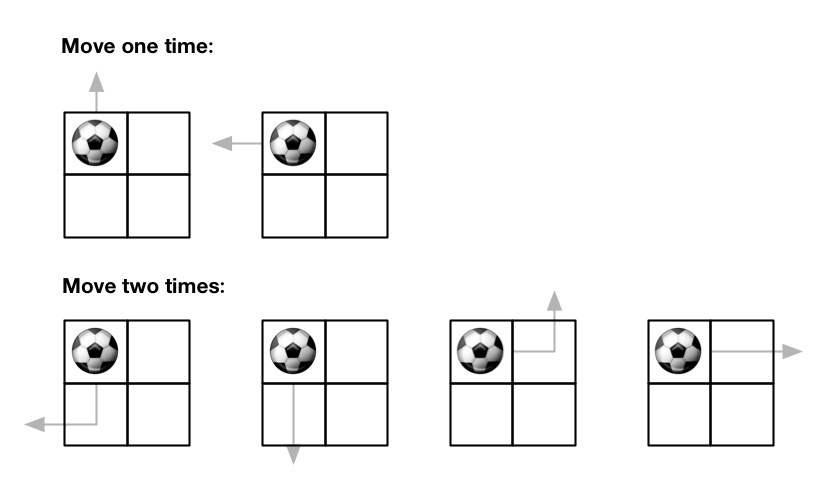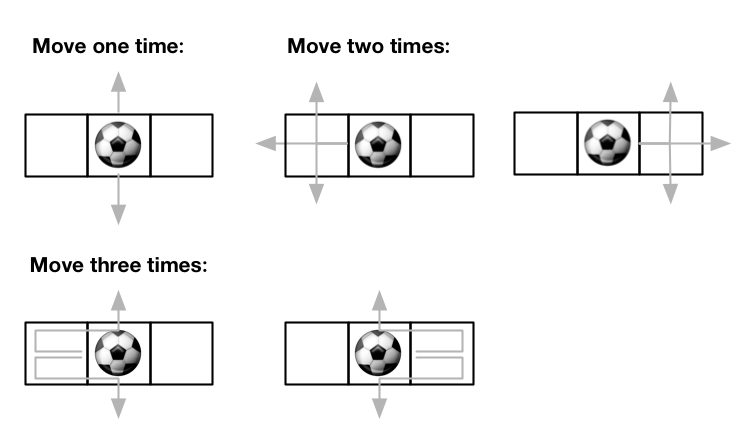GeetCode Hub

There is an m x n grid with a ball. The ball is initially at the position [startRow, startColumn]. You are allowed to move the ball to one of the four adjacent four cells in the grid (possibly out of the grid crossing the grid boundary). You can apply at most maxMove moves to the ball.

Given the five integers m, n, maxMove, startRow, startColumn, return the number of paths to move the ball out of the grid boundary. Since the answer can be very large, return it modulo 109 + 7.

Example 1:Input: m = 2, n = 2, maxMove = 2, startRow = 0, startColumn = 0
Output: 6

Example 2:Input: m = 1, n = 3, maxMove = 3, startRow = 0, startColumn = 1
Output: 12

Constraints:

• 1 <= m, n <= 50
• 0 <= maxMove <= 50
• 0 <= startRow <= m
• 0 <= startColumn <= n

class Solution { public int findPaths(int m, int n, int maxMove, int startRow, int startColumn) { } }